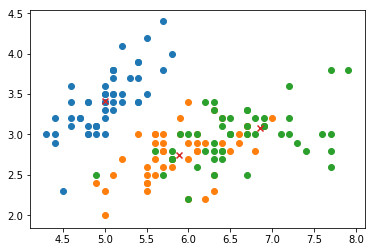2017年2月18日 K-means 原

K-means clustering aims to partition the samples into k sets so as to miniize the sum of distances between each pointer to the K center
The algorithm proceeds by iterating two steps: cluster assignment step and center update step, until assignments no longer change.

from __future__ import division
import numpy as np
from sklearn.cluster import KMeans
from sklearn.datasets import load_iris
import matplotlib.pyplot as plt
%matplotlib inline

X = data.data
y = data.target

class myKMean:

def compute_centers(self, X, predict, n_clusters):
centers = []
for c in range(n_clusters):
centers.append(np.mean(X[np.where(predict == c)], axis=0))

return np.array(centers)

def compute_cluster(self, X, centers):
predict = []
for x in X:
distances = [ np.sqrt(np.sum((x-c)**2)) for c in centers]
predict.append(np.argmin(distances))

return np.array(predict)

def fit(self, X, n_clusters):
predict = np.random.randint(n_clusters, size=X.shape)

while True:
self.centers = self.compute_centers(X, predict, n_clusters)
p = self.compute_cluster(X, self.centers)

if np.count_nonzero(predict - p) == 0:
break
else:
predict = p

def predict(self, X):
return self.compute_cluster(X, self.centers)

km = myKMean()
km.fit(X, len(np.unique(y)))

for i in range(data.target_names.shape):
Xi = X[np.where(y == i)]
plt.scatter(Xi[:,0], Xi[:,1])

plt.scatter(km.centers[:,0], km.centers[:,1], marker='x')2017年进九时间 2017进九的日子是12月22日，其实也就是“冬至日”。天文专家介绍说，“九九”是我国北方特别是黄河中下游地区更为适用的一种杂节气。 2017~2018年数九时间表 一九 2017年12月...

anlve
2018/01/27
0
0
Day186 | 遇见GCS（七）

7月份说超级节点计划马上开启，不过一个多月过去，还没有消息。 新闻：http://blockchain.game/news.html 开发者社区：http://developer.blockchain.game/ 新版官网上线：http://blockchain...

2018/08/21
0
0
Day172 | 遇见Mixin（十）

Mixin目前支持的链：EOS、BTC、BCH、ETH、ETC、LTC、XRP、SC。 XIN总量100万，50万作为节点激励，BigONE上IEO40万，白名单5万，团队5万，BigONE上的IEO有个大单拿走225000+。 XIN从诞生到现在...

2018/08/07
0
0
2016年1季度工作计划

imalexi
2016/01/28
1
0

jQuery EasyUI 1.5.x of insdep theme 1.0.0 正式版 更新截图请移至下方，附部分美化截图 关于 Insdep theme是基于EasyUI 1.5.x 的一款免费的美化主题包，拥有百度编辑器、cropper、Highcha...

magicweng
2017/02/13
9.8K
10

code-ortaerc
18分钟前
2
0

[TOC] 1.1.1标签操作v-text&v-html v-text：会把data中绑定的数据值原样输出。 v-html：会把data中值输出，且会自动解析html代码 <!--可以将指定的内容显示到标签体中--><标签 v-text=""></......

50分钟前
7
0
VMware vSphere的两种RDM磁盘

10
0
【AngularJS学习笔记】02 小杂烩及学习总结

14
0
Linux 内核的五大创新

18
0